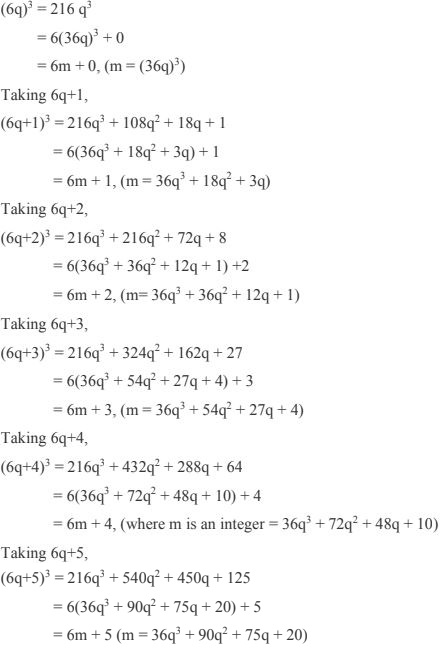# Chapter 1 Real Number NCERT Exemplar Solutions Exercise 1.4 Class 10 MathsChapter Name NCERT Maths Exemplar Solutions for Chapter 1 Real Numbers Exercise 1.4 Book Name NCERT Exemplar for Class 10 Maths Other Exercises Exercise 1.1Exercise 1.2Exercise 1.3 Related Study NCERT Solutions for Class 10 Maths

### Exercise 1.4 Solutions

1. Show that the cube of a positive integer of the form 6q + r, q is an integer and r = 0, 1, 2, 3, 4, 5 is also of the form 6m + r.

Solution

We have,
6q + r is a positive integer, where q is an integer and r = 0, 1, 2, 3, 4, 5
So, the positive integers are of the form 6q, 6q+1, 6q+2, 6q+3, 6q+4 and 6q+5.
Cube of these integers will be:
Taking 6q,Therefore, the cube of a positive integer of the form 6q + r, q is an integer and r = 0, 1, 2, 3, 4, 5 is also of the form 6m + r.

2. Prove that one and only one out of n, n + 2 and n + 4 is divisible by 3, where n is any positive integer.

Solution

Using Euclid's Algorithm,
Let the positive integer = n and b = 3.
n = 3q + r, where q is the quotient and r is the remainder.
Remainders can be 0, 1 and 2
So, n can be 3q, 3q + 1, 3q + 2
For n = 3q
n + 2 = 3q + 2
n + 4 = 3q + 4
In this case n is only divisible by 3.
For n = 3q + 1
n + 2 = 3q + 3
In this case n + 2 is divisible by  3.
For n = 3q + 2
n + 2 = 3q + 4
n + 4 = 3q + 2 + 4
= 3q + 6
In this case n + 4 is divisible by 3.
Therefore, we can say that one and only one out of n, n + 2 and n + 4 is divisible by 3.

3. Prove that one of any three consecutive positive integers must be divisible by 3.

Solution

Let the three consecutive number be n, n+1, n+2.
By Euclid’s algorithm, we have
n = 3q + r
Where q is quotient and r is remainder
The values of r can be = 0, 1, 2.
For r = 0,
n = 3q
n + 1 = 3q + 1
n+2 = 3q+2
So, here n is divisible by 3.
Now, For r = 1,
n = 3q+1
n+1=3q+2
n+2 = 3q+3
So, here n+2 is divisible by 3.
For r = 2,
n = 3q+2
n+1=3q+3
n+2 = 3q+4
So, here n+1 is divisible by 3.
Therefore one of any three consecutive positive integers must be divisible by 3.

4. For any positive integer n, prove that n3 – n is divisible by 6.

Solution

Let x = n3 – n
x = n(n2 – 1)
x = n(n – 1)(n + 1)
(n – 1), n, (n + 1) are consecutive integers so out of three consecutive numbers at least one will be even.
So, we can say that x is divisible by 2.
Sum of number = (n – 1) + n + (n + 1)
= n – 1 + n + n + 1
= 3n
Here, the sum of three consecutive numbers is divisible by 3, so one of them is divisible by 3.
So, out of n, (n – 1), (n + 1), one is divisible by 2 and one is divisible by 3 and
x = (n – 1) × n × (n + 1)
Hence, out of three factors of x, one is divisible by 2 and one is divisible by 3.
Therefore, x is divisible by 6 or n3 – n is divisible by 6.

5. Show that one and only one out of n, (n + 4), (n + 8), (n + 12), (n + 16) is divisible by 5, where n is any positive integer.
[Hint: Any positive integer can be written in the form 5q, (5q + 1), (5q + 2), (5q + 3), (5q +4)]

Solution
If a number n is divided by 5 then let the quotient is q and remainder is r. Then by Euclid’s algorithm,
n = 5q + r, where n, q, r are positive integers and 0 ≤ r < 5.
For, r = 0,
n = 5q + 0
= 5q
So, n is divisible by 5.
For, r = 1,
n = 5q + 1
n + 4 = (5q + 1) + 4
= 5q + 5
= 5(q + 1) divisible by 5.
We can say that (n + 4) is divisible by 5.
For r = 2,
n = 5q + 2
(n + 8) = (5q + 2) + 8
= 5q + 10 = 5(q + 2)
= 5m is divisible by 5.
We can say that, (n + 8) is divisible by 5.
For, r = 3,
n = 5q + 3
n + 12 = (5q + 3) + 12
= 5q + 15
= 5(q + 3) = 5m is divisible by 5.
We can say that, (n + 12) is divisible by 5.
For, r = 4,
n = 5q + 4
n + 16 = (5q + 4) + 16
= 5q + 20 = 5(q + 4)
(n + 16) = 5m is divisible by 5.
Therefore, n, (n + 4), (n + 8), (n + 12) and (n + 16) are divisible by 5.